﻿ MechDesigner Reference & User Interface > Dialogs > Dialog: Function-Block: Graph

# Dialog: Function-Block: Graph

Navigation:  MechDesigner Reference & User Interface > Dialogs >

# Dialog: Function-Block: Graph

## Graph FB

Use a Graph FB to plot Kinematic Motion-Data, Kinetostatic Force-Data and Cam-Data.

There are:

4 × Y-axis input: the top four(4) input-connectors

1 × X-axis input: the bottom input-connector is the X-axis for all of the graphs.

If you do not connect a wire to the X-axis input-connector, the X-axis of the graph is one machine-cycle, 0 – 360.

#### How to open the Graph InterfaceTo open the Graph FB dialog

 1.Double-click the Graph FB in the graphic-area. OR 1.

The Graph interface is now open.

### Graph Interface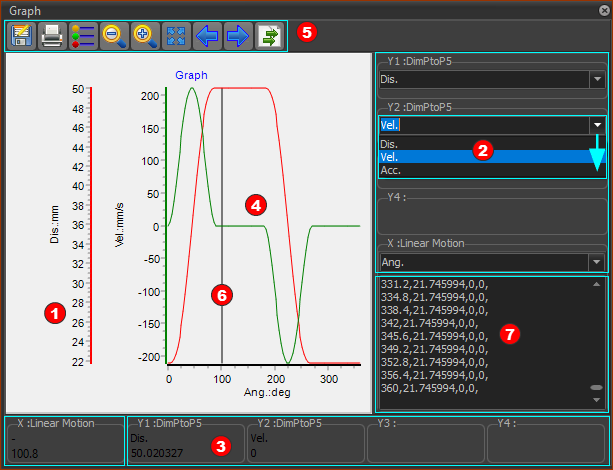Grap dialog/interface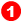Y–axes

 There is one Y-axis scale for each wire that you connect to an input-connector. Maximum = 4 From left to right, the Y-axis scales correspond to each wire that you connect to an input-connectors, from top to bottom. The color of each Y–axis scale is the same as the graph. 1.Edit the colors with the Graph Setting dialog. The minimum and maximum of the Y-axis scale are the minimum and maximum values of the data at the input-connector. 1.Edit the minimum and maximum values for the Y-axis scales with the Graph Setting dialog.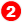Y–axis Data-Channels (Drop-Down):

 Each wire that you connect to an input-connector has 3 Data-Channels. 1.Click the drop-down arrow to select which Data-Channel to plot for each wire at the input-connector.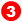Digital Readout (D.R.O.):

 The X–axis and the Y1 to Y4-axis values in the Digital Readout are those values of the graph at the position of the vertical cursor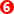The Number-Format for the X–axis and Y–axis values is configured in Application Settings | Number Format tab.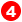Graph Area:

 There is a graph for each wire you connect to a Y-axis input-connector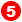Graph toolbar: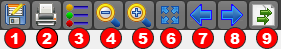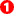Save data as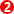Print graphs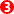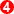Zoom-Out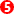Zoom-InZoom-Extents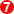Pan Left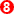Pan Right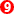Copy Graph Data to Clipboard – see below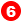Vertical-Cursor

 When you click or drag your pointer in the graph-area: •the Vertical-Cursor moves with your pointer •the Digital-Readoutsare equal to the Y-axis values of the Vertical-Cursor •the Master Machine Angle continuously updates to equal the X-axis value of the Vertical-Cursor •the kinematic-chains that are kinematically-defined move to agree with the Master Machine Angle.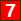Graph Data in Clipboard

 To list the graph data-values, 1.Click the right-most icon in the Graph toolbar. There is one data-point for each Machine Step - see Machine-Settings dialog > Number-of-Steps. You can Paste the data: •to Excel, or Notepad, ... •to the MotionDesigner Data-Transfer Table From the Data-Transfer Table, you can paste the data: •as a dumb Overlay-Trace to compare it with a motion •to a Position List, Acceleration List, or Z Raw Data segment-type, and link it to a Motion-Part or Motion-Point.

#### Y-Axis Data-Channels:

 Each wire that you connect to a Graph FB has three(3) data-channels. Use the drop-down arrow to select which Y-Axis Data-Channelto plot for each Y-axis. The Data-Channels that are available are a function of the Function-Block and wire you connect at the input to the Graph FB. Data-Channels: Kinematic Function-Blocks (Linear-Motion, Gearing, Motion, Motion-Dimension) you can plot: •Linear or angular position •Linear or angular velocity •Linear or angular acceleration Measurement FB or Point-Data FB you can plot: •Linear or angular position •Linear or angular velocity •Linear or angular acceleration Cam-Data FB •Pressure Angle. You can plot three Pressure Angles – See Pressure Angle •Radius-of-Curvature. You can plot the Radii-of-Curvature of the Inner and the Outer Cam. •Contact Cam Force •Maximum Contact Shear Stress. •Sliding-Velocity Plot the Sliding-Velocity between the Cam-Profile and Cam-Follower for the Inner Cam and the Outer Cam. Force-Data FB The output form a Force-Data FB is a function of the element with which it is linked. If you link a Force-Data FB to a: ŸPin-Joint with a Motor, you can plot the Application Load. ŸSlide-Joint with a Motor, you can plot the Application Load ŸJoint that does not have a Motor, you can plot: ☑Total Force ( FT = √(FX2 + FY2) ☑Force along the X-coordinate of a Point: FX ☑Force along the Y-coordinate of a Point: FY Ÿ2D-Cam: you can plot the Cam Contact Force ŸSpring FB: you can plot the Total Force, X Force, Y Force acting on the Anchor-Point.

#### Why are the Y–axis values in the DROdifferent to the vector-values in the main graphic-area?

 The values may be slightly different because, in the graph, the X–axis and Y–axis values at the D.R.O. must jump from 'machine -step' to 'machine-step'. See Machine Settings dialog > Number-of-Steps. In the graphic-area, the vector values are calculated for the value of the Master Machine Angle, which may not coincide with a machine step.